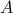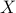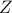# Description of linfilter.m

Matlab syntax
```>> [Af,Bf,Cf,Df] = linfilter(A,X,Z);
```

Matlab function linfilter.m takes the linear dynamical generatorand the correlation matrices problem dataandwhich results from the function ccama.m and returns the state-space realization of the linear filter which generates the suitable colored-in-time forcing into the linear dynamics.

### linfilter.m

linfilter
```% Filter realization
%
% Written by Armin Zare and Mihailo Jovanovic, April 2016
%
```
```function [Af,Bf,Cf,Df] = linfilter(A,X,Z)

% Spectal decomposition
% B is the input matrix; H is the cross-correlation of state and forcing
[B, H, S] = Qdecomposition(Z);

% nb is the rank of B or required nubmer of input channels
[mb, nb] = size(B);

% Rho, covariance of white noise
Rho = eye(nb);

% Filter realization
Cf = (-.5*Rho*B'+ H')/X;
Af = A + B*Cf;
Bf = B;
Df = eye(nb);

end
```